This book is archived and will be removed July 6, 2022. Please use the updated version.

Capacitance

# 49 Capacitors and Capacitance

### Learning Objectives

By the end of this section, you will be able to:

• Explain the concepts of a capacitor and its capacitance
• Describe how to evaluate the capacitance of a system of conductors

A capacitor is a device used to store electrical charge and electrical energy. It consists of at least two electrical conductors separated by a distance. (Note that such electrical conductors are sometimes referred to as “electrodes,” but more correctly, they are “capacitor plates.”) The space between capacitors may simply be a vacuum, and, in that case, a capacitor is then known as a “vacuum capacitor.” However, the space is usually filled with an insulating material known as a dielectric. (You will learn more about dielectrics in the sections on dielectrics later in this chapter.) The amount of storage in a capacitor is determined by a property called capacitance, which you will learn more about a bit later in this section.

Capacitors have applications ranging from filtering static from radio reception to energy storage in heart defibrillators. Typically, commercial capacitors have two conducting parts close to one another but not touching, such as those in (Figure). Most of the time, a dielectric is used between the two plates. When battery terminals are connected to an initially uncharged capacitor, the battery potential moves a small amount of charge of magnitude Q from the positive plate to the negative plate. The capacitor remains neutral overall, but with charges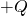and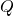residing on opposite plates.

Both capacitors shown here were initially uncharged before being connected to a battery. They now have charges ofand(respectively) on their plates. (a) A parallel-plate capacitor consists of two plates of opposite charge with area A separated by distance d. (b) A rolled capacitor has a dielectric material between its two conducting sheets (plates).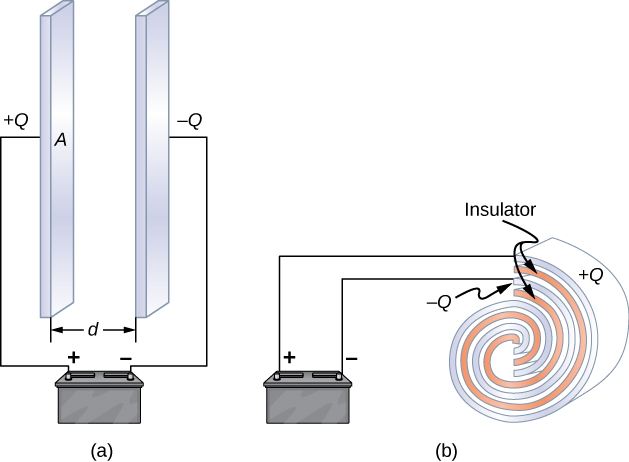A system composed of two identical parallel-conducting plates separated by a distance is called a parallel-plate capacitor ((Figure)). The magnitude of the electrical field in the space between the parallel plates is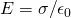, where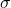denotes the surface charge density on one plate (recall thatis the charge Q per the surface area A). Thus, the magnitude of the field is directly proportional to Q.

The charge separation in a capacitor shows that the charges remain on the surfaces of the capacitor plates. Electrical field lines in a parallel-plate capacitor begin with positive charges and end with negative charges. The magnitude of the electrical field in the space between the plates is in direct proportion to the amount of charge on the capacitor.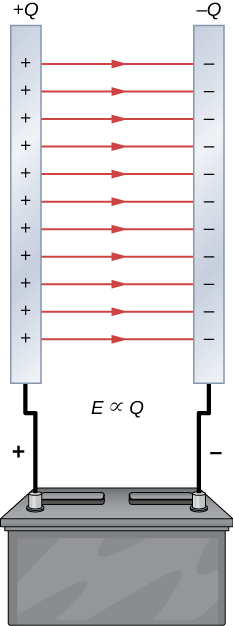Capacitors with different physical characteristics (such as shape and size of their plates) store different amounts of charge for the same applied voltage V across their plates. The capacitance C of a capacitor is defined as the ratio of the maximum charge Q that can be stored in a capacitor to the applied voltage V across its plates. In other words, capacitance is the largest amount of charge per volt that can be stored on the device: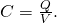The SI unit of capacitance is the farad (F), named after Michael Faraday (1791–1867). Since capacitance is the charge per unit voltage, one farad is one coulomb per one volt, or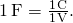By definition, a 1.0-F capacitor is able to store 1.0 C of charge (a very large amount of charge) when the potential difference between its plates is only 1.0 V. One farad is therefore a very large capacitance. Typical capacitance values range from picofarads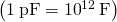to millifarads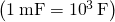, which also includes microfarads (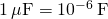). Capacitors can be produced in various shapes and sizes ((Figure)).

These are some typical capacitors used in electronic devices. A capacitor’s size is not necessarily related to its capacitance value. (credit: Windell Oskay)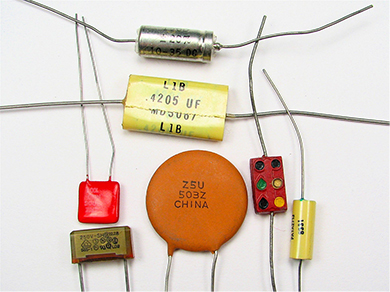### Calculation of Capacitance

We can calculate the capacitance of a pair of conductors with the standard approach that follows.

Problem-Solving Strategy: Calculating Capacitance
1. Assume that the capacitor has a charge Q.
2. Determine the electrical field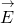between the conductors. If symmetry is present in the arrangement of conductors, you may be able to use Gauss’s law for this calculation.
3. Find the potential difference between the conductors from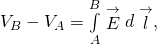where the path of integration leads from one conductor to the other. The magnitude of the potential difference is then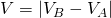.

4. With V known, obtain the capacitance directly from (Figure).

To show how this procedure works, we now calculate the capacitances of parallel-plate, spherical, and cylindrical capacitors. In all cases, we assume vacuum capacitors (empty capacitors) with no dielectric substance in the space between conductors.

### Parallel-Plate Capacitor

The parallel-plate capacitor ((Figure)) has two identical conducting plates, each having a surface area A, separated by a distance d. When a voltage V is applied to the capacitor, it stores a charge Q, as shown. We can see how its capacitance may depend on A and d by considering characteristics of the Coulomb force. We know that force between the charges increases with charge values and decreases with the distance between them. We should expect that the bigger the plates are, the more charge they can store. Thus, C should be greater for a larger value of A. Similarly, the closer the plates are together, the greater the attraction of the opposite charges on them. Therefore, C should be greater for a smaller d.

In a parallel-plate capacitor with plates separated by a distance d, each plate has the same surface area A.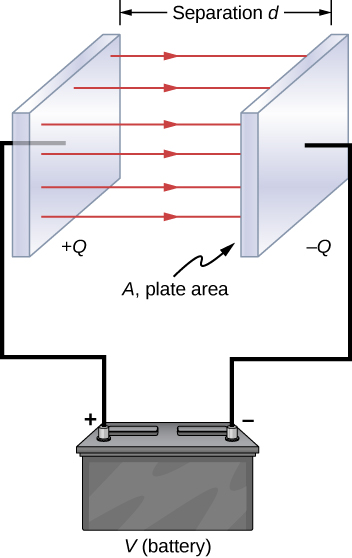We define the surface charge densityon the plates as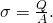We know from previous chapters that when d is small, the electrical field between the plates is fairly uniform (ignoring edge effects) and that its magnitude is given by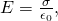where the constant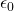is the permittivity of free space,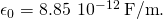The SI unit of F/m is equivalent to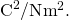Since the electrical fieldbetween the plates is uniform, the potential difference between the plates is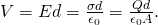Therefore (Figure) gives the capacitance of a parallel-plate capacitor as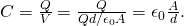Notice from this equation that capacitance is a function only of the geometry and what material fills the space between the plates (in this case, vacuum) of this capacitor. In fact, this is true not only for a parallel-plate capacitor, but for all capacitors: The capacitance is independent of Q or V. If the charge changes, the potential changes correspondingly so that Q/V remains constant.

Capacitance and Charge Stored in a Parallel-Plate Capacitor (a) What is the capacitance of an empty parallel-plate capacitor with metal plates that each have an area of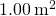, separated by 1.00 mm? (b) How much charge is stored in this capacitor if a voltage of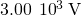is applied to it?

Strategy Finding the capacitance C is a straightforward application of (Figure). Once we find C, we can find the charge stored by using (Figure).

Solution

1. Entering the given values into (Figure) yields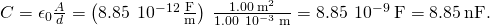This small capacitance value indicates how difficult it is to make a device with a large capacitance.

2. Inverting (Figure) and entering the known values into this equation gives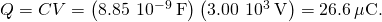Significance This charge is only slightly greater than those found in typical static electricity applications. Since air breaks down (becomes conductive) at an electrical field strength of about 3.0 MV/m, no more charge can be stored on this capacitor by increasing the voltage.

A 1-F Parallel-Plate Capacitor Suppose you wish to construct a parallel-plate capacitor with a capacitance of 1.0 F. What area must you use for each plate if the plates are separated by 1.0 mm?

Solution Rearranging (Figure), we obtain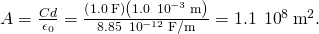Each square plate would have to be 10 km across. It used to be a common prank to ask a student to go to the laboratory stockroom and request a 1-F parallel-plate capacitor, until stockroom attendants got tired of the joke.

Check Your Understanding The capacitance of a parallel-plate capacitor is 2.0 pF. If the area of each plate is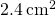, what is the plate separation?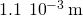Check Your Understanding Verify that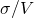and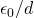have the same physical units.

### Spherical Capacitor

A spherical capacitor is another set of conductors whose capacitance can be easily determined ((Figure)). It consists of two concentric conducting spherical shells of radii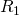(inner shell) and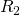(outer shell). The shells are given equal and opposite chargesand, respectively. From symmetry, the electrical field between the shells is directed radially outward. We can obtain the magnitude of the field by applying Gauss’s law over a spherical Gaussian surface of radius r concentric with the shells. The enclosed charge is; therefore we have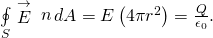Thus, the electrical field between the conductors is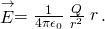We substitute thisinto (Figure) and integrate along a radial path between the shells: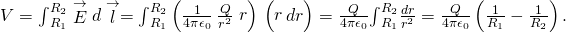In this equation, the potential difference between the plates is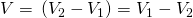. We substitute this result into (Figure) to find the capacitance of a spherical capacitor: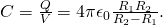A spherical capacitor consists of two concentric conducting spheres. Note that the charges on a conductor reside on its surface.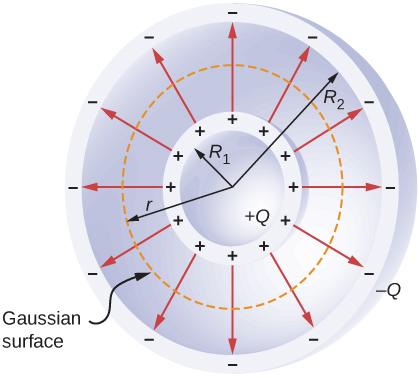Capacitance of an Isolated Sphere Calculate the capacitance of a single isolated conducting sphere of radiusand compare it with (Figure) in the limit as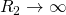.

Strategy We assume that the charge on the sphere is Q, and so we follow the four steps outlined earlier. We also assume the other conductor to be a concentric hollow sphere of infinite radius.

Solution On the outside of an isolated conducting sphere, the electrical field is given by (Figure). The magnitude of the potential difference between the surface of an isolated sphere and infinity is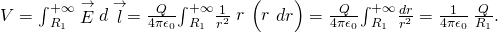The capacitance of an isolated sphere is therefore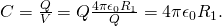Significance The same result can be obtained by taking the limit of (Figure) as. A single isolated sphere is therefore equivalent to a spherical capacitor whose outer shell has an infinitely large radius.

Check Your Understanding The radius of the outer sphere of a spherical capacitor is five times the radius of its inner shell. What are the dimensions of this capacitor if its capacitance is 5.00 pF?

3.59 cm, 17.98 cm

### Cylindrical Capacitor

A cylindrical capacitor consists of two concentric, conducting cylinders ((Figure)). The inner cylinder, of radius, may either be a shell or be completely solid. The outer cylinder is a shell of inner radius. We assume that the length of each cylinder is l and that the excess chargesandreside on the inner and outer cylinders, respectively.

A cylindrical capacitor consists of two concentric, conducting cylinders. Here, the charge on the outer surface of the inner cylinder is positive (indicated by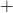) and the charge on the inner surface of the outer cylinder is negative (indicated by).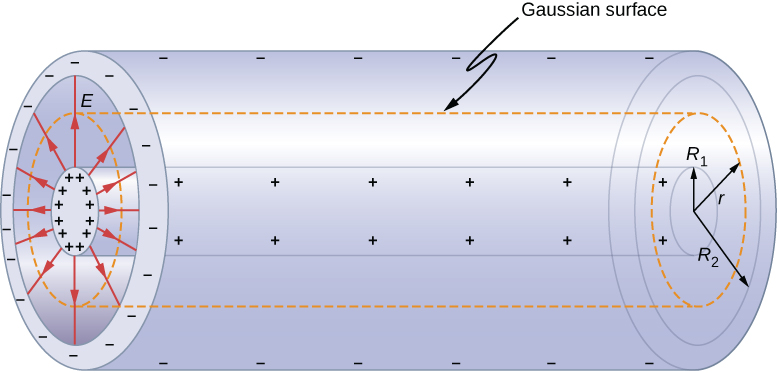With edge effects ignored, the electrical field between the conductors is directed radially outward from the common axis of the cylinders. Using the Gaussian surface shown in (Figure), we have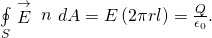Therefore, the electrical field between the cylinders is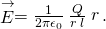Here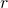is the unit radial vector along the radius of the cylinder. We can substitute into (Figure) and find the potential difference between the cylinders: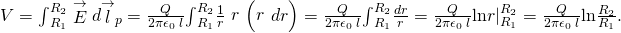Thus, the capacitance of a cylindrical capacitor is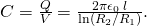As in other cases, this capacitance depends only on the geometry of the conductor arrangement. An important application of (Figure) is the determination of the capacitance per unit length of a coaxial cable, which is commonly used to transmit time-varying electrical signals. A coaxial cable consists of two concentric, cylindrical conductors separated by an insulating material. (Here, we assume a vacuum between the conductors, but the physics is qualitatively almost the same when the space between the conductors is filled by a dielectric.) This configuration shields the electrical signal propagating down the inner conductor from stray electrical fields external to the cable. Current flows in opposite directions in the inner and the outer conductors, with the outer conductor usually grounded. Now, from (Figure), the capacitance per unit length of the coaxial cable is given by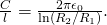In practical applications, it is important to select specific values of C/l. This can be accomplished with appropriate choices of radii of the conductors and of the insulating material between them.

Check Your Understanding When a cylindrical capacitor is given a charge of 0.500 nC, a potential difference of 20.0 V is measured between the cylinders. (a) What is the capacitance of this system? (b) If the cylinders are 1.0 m long, what is the ratio of their radii?

a. 25.0 pF; b. 9.2

Several types of practical capacitors are shown in (Figure). Common capacitors are often made of two small pieces of metal foil separated by two small pieces of insulation (see (Figure)(b)). The metal foil and insulation are encased in a protective coating, and two metal leads are used for connecting the foils to an external circuit. Some common insulating materials are mica, ceramic, paper, and Teflon™ non-stick coating.

Another popular type of capacitor is an electrolytic capacitor. It consists of an oxidized metal in a conducting paste. The main advantage of an electrolytic capacitor is its high capacitance relative to other common types of capacitors. For example, capacitance of one type of aluminum electrolytic capacitor can be as high as 1.0 F. However, you must be careful when using an electrolytic capacitor in a circuit, because it only functions correctly when the metal foil is at a higher potential than the conducting paste. When reverse polarization occurs, electrolytic action destroys the oxide film. This type of capacitor cannot be connected across an alternating current source, because half of the time, ac voltage would have the wrong polarity, as an alternating current reverses its polarity (see Alternating-Current Circuts on alternating-current circuits).

A variable air capacitor ((Figure)) has two sets of parallel plates. One set of plates is fixed (indicated as “stator”), and the other set of plates is attached to a shaft that can be rotated (indicated as “rotor”). By turning the shaft, the cross-sectional area in the overlap of the plates can be changed; therefore, the capacitance of this system can be tuned to a desired value. Capacitor tuning has applications in any type of radio transmission and in receiving radio signals from electronic devices. Any time you tune your car radio to your favorite station, think of capacitance.

In a variable air capacitor, capacitance can be tuned by changing the effective area of the plates. (credit: modification of work by Robbie Sproule)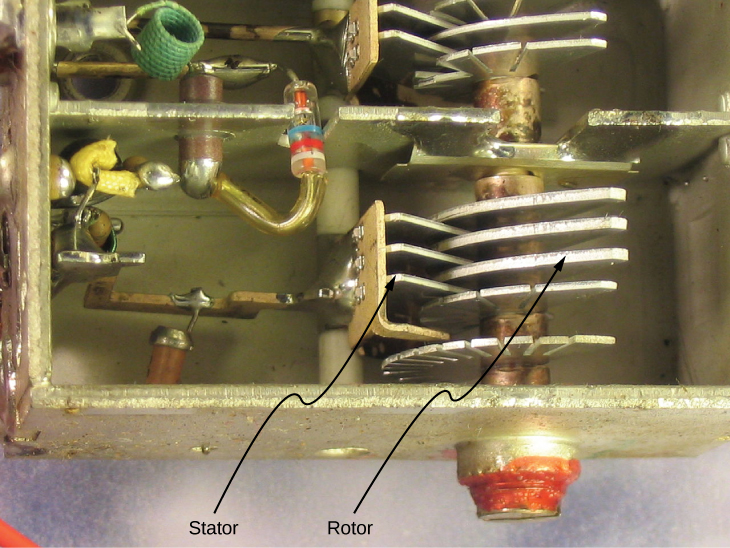The symbols shown in (Figure) are circuit representations of various types of capacitors. We generally use the symbol shown in (Figure)(a). The symbol in (Figure)(c) represents a variable-capacitance capacitor. Notice the similarity of these symbols to the symmetry of a parallel-plate capacitor. An electrolytic capacitor is represented by the symbol in part (Figure)(b), where the curved plate indicates the negative terminal.

This shows three different circuit representations of capacitors. The symbol in (a) is the most commonly used one. The symbol in (b) represents an electrolytic capacitor. The symbol in (c) represents a variable-capacitance capacitor.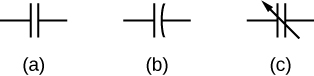An interesting applied example of a capacitor model comes from cell biology and deals with the electrical potential in the plasma membrane of a living cell ((Figure)). Cell membranes separate cells from their surroundings but allow some selected ions to pass in or out of the cell. The potential difference across a membrane is about 70 mV. The cell membrane may be 7 to 10 nm thick. Treating the cell membrane as a nano-sized capacitor, the estimate of the smallest electrical field strength across its ‘plates’ yields the value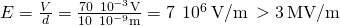.

This magnitude of electrical field is great enough to create an electrical spark in the air.

The semipermeable membrane of a biological cell has different concentrations of ions on its interior surface than on its exterior. Diffusion moves the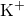(potassium) and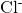(chloride) ions in the directions shown, until the Coulomb force halts further transfer. In this way, the exterior of the membrane acquires a positive charge and its interior surface acquires a negative charge, creating a potential difference across the membrane. The membrane is normally impermeable to Na+ (sodium ions).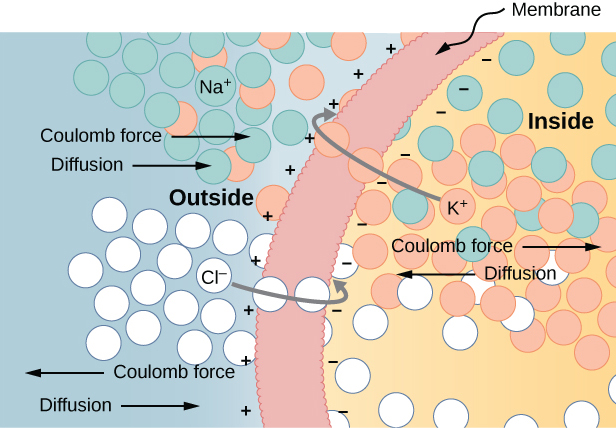Visit the PhET Explorations: Capacitor Lab to explore how a capacitor works. Change the size of the plates and add a dielectric to see the effect on capacitance. Change the voltage and see charges built up on the plates. Observe the electrical field in the capacitor. Measure the voltage and the electrical field.

### Summary

• A capacitor is a device that stores an electrical charge and electrical energy. The amount of charge a vacuum capacitor can store depends on two major factors: the voltage applied and the capacitor’s physical characteristics, such as its size and geometry.
• The capacitance of a capacitor is a parameter that tells us how much charge can be stored in the capacitor per unit potential difference between its plates. Capacitance of a system of conductors depends only on the geometry of their arrangement and physical properties of the insulating material that fills the space between the conductors. The unit of capacitance is the farad, where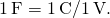### Conceptual Questions

Does the capacitance of a device depend on the applied voltage? Does the capacitance of a device depend on the charge residing on it?

no; yes

Would you place the plates of a parallel-plate capacitor closer together or farther apart to increase their capacitance?

The value of the capacitance is zero if the plates are not charged. True or false?

false

If the plates of a capacitor have different areas, will they acquire the same charge when the capacitor is connected across a battery?

Does the capacitance of a spherical capacitor depend on which sphere is charged positively or negatively?

no

### Problems

What charge is stored in a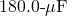capacitor when 120.0 V is applied to it?

21.6 mC

Find the charge stored when 5.50 V is applied to an 8.00-pF capacitor.

Calculate the voltage applied to a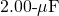capacitor when it holds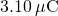of charge.

1.55 V

What voltage must be applied to an 8.00-nF capacitor to store 0.160 mC of charge?

What capacitance is needed to store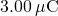of charge at a voltage of 120 V?

25.0 nF

What is the capacitance of a large Van de Graaff generator’s terminal, given that it stores 8.00 mC of charge at a voltage of 12.0 MV?

The plates of an empty parallel-plate capacitor of capacitance 5.0 pF are 2.0 mm apart. What is the area of each plate?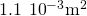A 60.0-pF vacuum capacitor has a plate area of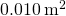. What is the separation between its plates?

A set of parallel plates has a capacitance of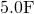. How much charge must be added to the plates to increase the potential difference between them by 100 V?

500 µC

Consider Earth to be a spherical conductor of radius 6400 km and calculate its capacitance.

If the capacitance per unit length of a cylindrical capacitor is 20 pF/m, what is the ratio of the radii of the two cylinders?

1:16

An empty parallel-plate capacitor has a capacitance of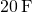. How much charge must leak off its plates before the voltage across them is reduced by 100 V?

### Glossary

capacitance
amount of charge stored per unit volt
capacitor
device that stores electrical charge and electrical energy
dielectric
insulating material used to fill the space between two plates
parallel-plate capacitor
system of two identical parallel conducting plates separated by a distance